﻿ 考虑参考价格效应的两期闭环供应链Nash均衡博弈 Nash Game in a Two-Period Closed-Loop Supply Chain with Reference Price Effect

Vol. 08  No. 06 ( 2019 ), Article ID: 30903 , 10 pages
10.12677/ASS.2019.86143

Nash Game in a Two-Period Closed-Loop Supply Chain with Reference Price Effect

Hao Sun, Zhuang Sui

School of Business, Qingdao University, Qingdao Shandong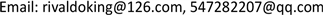Received: Jun. 4th, 2019; accepted: Jun. 17th, 2019; published: Jun. 24th, 2019ABSTRACT

Nash game models in a two-period closed-loop supply chain (CLSC) system with reference price effect are established under three different kinds of collection modes respectively: manufacturer-collection, retailer-collection and third-party-collection. The equilibrium prices, collection rates and channel member profits under three modes are derived and compared. Then the impacts of reference price effect on CLSC are analyzed. The conclusions show that: manufacturer-collection mode can maximize the environmental performance and the total profit of CLSC, but the manufacturer cannot realize his own profit maximization. The comparison between retailer-collection and third-party-collection depends on the collection subsidy rate. The reference price effect has a positive impact on the first-period price and the second-period demand in all three modes, while its impact on the second-period price depends upon the collection scale parameter. The increase of the reference price effect discourages the collection enthusiasm of the collector. All the channel members can benefit from the reference price effect.

Keywords:Two-Period, Closed Loop Supply Chain, Nash Equilibrium, Reference Price Effect1. 引言

2. 模型假设和参数定义

2.1. 问题描述和相关假设

2.2. 参变量说明

$B>\frac{2\left(1+\theta \right)\left(\Delta -g\right)\left[\alpha -c+\Delta -g\right]}{4+4\theta -{\theta }^{2}}.$

3. 不同回收模式下两期闭环供应链定价与生产再制造策略

3.1. 制造商回收

${\pi }_{\text{M}}^{\text{M}}\left({w}_{1},{w}_{2},\tau \right)=\left[\left({w}_{1}-c\right){q}_{1}-B{\tau }^{2}/2\right]+\left({w}_{2}-c\right){q}_{2}+\left(\Delta -g\right)\tau {q}_{1}$ (1)

${\pi }_{\text{R}}^{\text{M}}\left({p}_{1},{p}_{2}\right)=\left({p}_{1}-{w}_{1}\right){q}_{1}+\left({p}_{2}-{w}_{2}\right){q}_{2}$ (2)

${\pi }_{\text{M2}}^{\text{M}}\left({w}_{2}\right)=\left({w}_{2}-c\right)\left[\alpha -{p}_{2}-\theta \left({p}_{2}-{p}_{1}\right)\right]+\left(\Delta -g\right)\tau {q}_{1}$ (3)

${\pi }_{\text{R2}}^{\text{M}}\left({p}_{2}\right)=\left({p}_{2}-{w}_{2}\right)\left[\alpha -{p}_{2}-\theta \left({p}_{2}-{p}_{1}\right)\right]$ (4)

${w}_{\text{2}}^{\text{M}}\left({p}_{\text{1}}^{\text{M}}\right)=\frac{\alpha +\theta {p}_{1}}{3\left(1+\theta \right)}+\frac{2c}{3}$ (5)

${p}_{\text{2}}^{\text{M}}\left({p}_{\text{1}}^{\text{M}}\right)=\frac{2\left(\alpha +\theta {p}_{\text{1}}^{\text{M}}\right)}{3\left(1+\theta \right)}+\frac{c}{3}$ (6)

${p}_{\text{1}}^{\text{M}\ast }=\alpha -\frac{\left(1+\theta \right)\Lambda B}{XB-9{\Phi }_{\text{M}}}$

${\tau }^{\text{M}\ast }=\frac{\left(1+\theta \right)\left(\Delta -g\right)\Lambda }{XB-9{\Phi }_{\text{M}}}$

${w}_{\text{1}}^{\text{M}\ast }=\frac{\left(9-2\theta \right)\alpha +2\theta c}{9}-\frac{2\left(9+9\theta -{\theta }^{2}\right)\Lambda B}{9\left(XB-9{\Phi }_{\text{M}}\right)}$

3.2. 零 售商回收

${\pi }_{\text{M}}^{\text{R}}\left({w}_{1},{w}_{2}\right)=\left({w}_{1}-c\right){q}_{1}+\left({w}_{2}-c\right){q}_{2}+\left[\Delta -\left(1+\mu \right)g\right]\tau {q}_{1}$ (7)

$\begin{array}{l}{\pi }_{\text{R}}^{\text{R}}\left({p}_{1},{p}_{2},\tau \right)=\left[\left({p}_{1}-{w}_{1}\right){q}_{1}-B{\tau }^{2}/2\right]\\ \text{\hspace{0.17em}}\text{\hspace{0.17em}}\text{\hspace{0.17em}}\text{\hspace{0.17em}}\text{\hspace{0.17em}}\text{\hspace{0.17em}}\text{\hspace{0.17em}}\text{\hspace{0.17em}}\text{\hspace{0.17em}}\text{\hspace{0.17em}}\text{\hspace{0.17em}}\text{\hspace{0.17em}}\text{\hspace{0.17em}}\text{\hspace{0.17em}}\text{\hspace{0.17em}}\text{\hspace{0.17em}}\text{\hspace{0.17em}}\text{\hspace{0.17em}}\text{\hspace{0.17em}}+\left[\left({p}_{2}-{w}_{2}\right){q}_{2}+\mu g\tau {q}_{1}\right]\end{array}$ (8)

${p}_{\text{1}}^{\text{R}\ast }=\alpha -\frac{\left(1+\theta \right)\Lambda B}{XB-9{\Phi }_{\text{R}}}$

${w}_{\text{1}}^{\text{R*}}=\frac{\left(9-2\theta \right)\alpha +2\theta c}{9}-\frac{\left[2\left(9+9\theta -{\theta }^{2}\right)B-9\left(1+\theta \right){\left(\mu g\right)}^{2}\right]\Lambda }{9\left(XB-9{\Phi }_{\text{R}}\right)}$

${\tau }^{\text{R}\ast }=\frac{\left(1+\theta \right)\mu g\Lambda }{XB-9{\Phi }_{\text{R}}}$

3.3. 第三方回收

${\pi }_{\text{M}}^{\text{T}}\left({w}_{1},{w}_{2}\right)=\left({w}_{1}-c\right){q}_{1}+\left({w}_{2}-c\right){q}_{2}+\left[\Delta -\left(1+\mu \right)g\right]\tau {q}_{1}$ (9)

${\pi }_{\text{R}}^{\text{T}}\left({p}_{1},{p}_{2}\right)=\left({p}_{1}-{w}_{1}\right){q}_{1}+\left({p}_{2}-{w}_{2}\right){q}_{2}$ (10)

${\pi }_{\text{T}}^{\text{T}}\left(\tau \right)=-B{\tau }^{2}/2+\mu g\tau {q}_{1}$ (11)

${w}_{\text{1}}^{\text{T}\ast }=\frac{\left(9-2\theta \right)\alpha +2\theta c}{9}-\frac{2\left(9+9\theta -{\theta }^{2}\right)\Lambda B}{9\left(XB-9{\Phi }_{\text{T}}\right)}$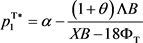4. 均衡解的性质及比较分析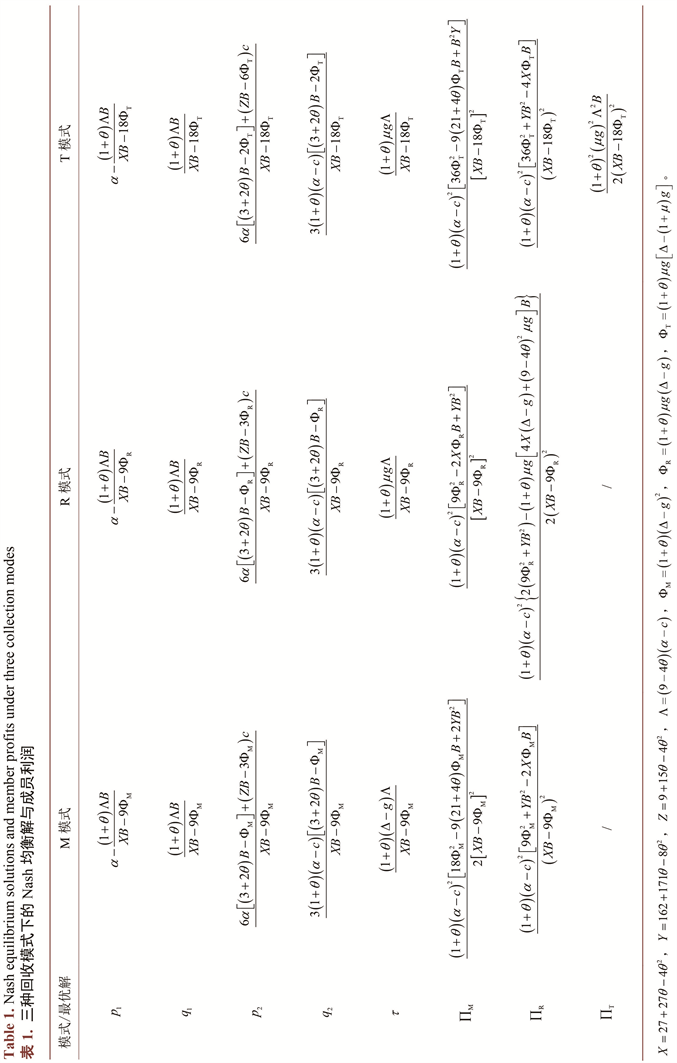1) $\partial {p}_{1}^{\text{M}\ast }/\partial \theta >\text{0}$$\partial {q}_{\text{1}}^{\text{M*}}/\partial \theta <\text{0}$$\partial {q}_{\text{2}}^{\text{M}\ast }/\partial \theta >\text{0}$$\partial {p}_{1}^{\text{R}\ast }/\partial \theta >\text{0}$$\partial {q}_{\text{1}}^{\text{R*}}/\partial \theta <\text{0}$$\partial {q}_{\text{2}}^{\text{R}\ast }/\partial \theta >\text{0}$$\partial {p}_{1}^{\text{T}\ast }/\partial \theta >\text{0}$$\partial {q}_{\text{1}}^{\text{T*}}/\partial \theta <\text{0}$$\partial {q}_{\text{2}}^{\text{T}\ast }/\partial \theta >\text{0}$

2) 当 $B>\kappa \cdot {\Phi }_{\text{M}}$ 时， $\partial {p}_{\text{2}}^{\text{M}\ast }/\partial \theta >\text{0}$，反之当 $B\le \kappa \cdot {\Phi }_{\text{M}}$ 时， $\partial {p}_{\text{2}}^{\text{M}\ast }/\partial \theta <\text{0}$；当 $B>\kappa \cdot {\Phi }_{\text{R}}$ 时， $\partial {p}_{\text{2}}^{\text{R*}}/\partial \theta >\text{0}$，反之当 $B\le \kappa \cdot {\Phi }_{\text{R}}$ 时， $\partial {p}_{\text{2}}^{\text{R*}}/\partial \theta <\text{0}$；当 $B>\kappa \cdot {\Phi }_{\text{T}}$ 时， $\partial {p}_{\text{2}}^{\text{T*}}/\partial \theta >\text{0}$，反之当 $B\le \kappa \cdot {\Phi }_{\text{T}}$ 时， $\partial {p}_{\text{2}}^{\text{T*}}/\partial \theta <\text{0}$。其中 $\kappa =\frac{9-8\theta -4{\theta }^{2}}{\left(1+\theta \right)\left(27-24\theta -8{\theta }^{2}\right)}$

3) $\partial {\tau }^{\text{M}\ast }/\partial \theta <\text{0}$$\partial {\tau }^{\text{R}\ast }/\partial \theta <\text{0}$$\partial {\tau }^{\text{T}\ast }/\partial \theta <\text{0}$

4) $\partial {\pi }_{\text{M}}^{\text{M}\ast }/\partial \theta >\text{0}$$\partial {\pi }_{\text{R}}^{\text{M}\ast }/\partial \theta >\text{0}$$\partial {\pi }^{\text{M}\ast }/\partial \theta >\text{0}$$\partial {\pi }_{\text{M}}^{\text{R}\ast }/\partial \theta >\text{0}$$\partial {\pi }_{\text{R}}^{\text{R*}}/\partial \theta >\text{0}$$\partial {\pi }^{\text{R}\ast }/\partial \theta >\text{0}$$\partial {\pi }_{\text{M}}^{\text{T}\ast }/\partial \theta >\text{0}$$\partial {\pi }_{\text{R}}^{\text{T*}}/\partial \theta >\text{0}$$\partial {\pi }_{\text{T}}^{\text{T*}}/\partial \theta >\text{0}$$\partial {\pi }^{\text{T}\ast }/\partial \theta >\text{0}$

$B>\frac{2\left(1+\theta \right)\left(\Delta -g\right)\left[\alpha -c+\Delta -g\right]}{4+4\theta -{\theta }^{2}}>\frac{2{\Phi }_{\text{M}}}{4+4\theta -{\theta }^{2}}$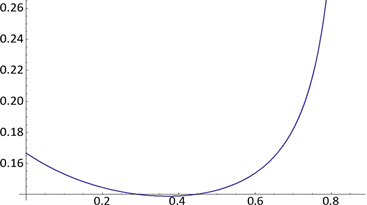(a)

$0<\theta \le 3\left(\sqrt{10}-2\right)/4$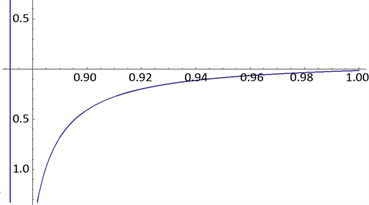(b)

$3\left(\sqrt{10}-2\right)/4<\theta <1$

Figure 1. The image of the function $F\left(\theta \right)$ in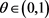interval

5. 结束语

1) 在Nash均衡博弈情形下，相比于零售商回收和第三方回收，制造商承担回收工作能实现闭环供应链系统利润最大化，也更能促进环保绩效的提升，此外较低的价格对消费者也有利。但该模式下制造商无法获得最高收益。

2) 在Nash均衡博弈情形下，从回收绩效与系统利润视角，零售商回收与第三方回收的优劣取决于制造商提供给回收方的成本补贴，当成本补贴率高于阈值 $\left(\Delta -g\right)/2g$ 时，零售商回收优于第三方回收；相反当成本补贴率低于该阈值时，则是第三方回收更优。但无论成本补贴率如何变化，零售商都不具备从事回收工作的动机。

3) 在Nash均衡博弈情形下，随着参考价格因子的增加，三种分散式回收模式的第一期价格和销量分别提高和降低，第二期销量均提高，而第二期价格取决于回收规模参数的取值；回收率均降低；所有渠道成员和闭环供应链系统均能从参考价格效应中获益。

Nash Game in a Two-Period Closed-Loop Supply Chain with Reference Price Effect[J]. 社会科学前沿, 2019, 08(06): 1033-1042. https://doi.org/10.12677/ASS.2019.86143

1. 1. Ferrer, G. (1997) The Economics of Tire Remanufacturing. Resources, Conservation and Recycling, 19, 221-255. https://doi.org/10.1016/S0921-3449(96)01181-0

2. 2. 李新军, 达庆利. 基于产品寿命次数的闭环供应链生产优化问题研究[J]. 管理工程学报, 2009, 23(2): 50-54.

3. 3. Ferrer, G. and Swaminathan, J.M. (2006) Managing New and Remanufactured Products. Management Science, 52, 15-26. https://doi.org/10.1287/mnsc.1050.0465

4. 4. Ferrer, G. and Swaminathan, J.M. (2010) Managing New and Differentiated Remanufactured Products. European Journal of Operational Research, 203, 370-379. https://doi.org/10.1016/j.ejor.2009.08.007

5. 5. Bulmuş, S.C., Zhu, S.X. and Teunter, R. (2013) Capacity and Production Decisions under a Remanufacturing Strategy. International Journal of Production Economics, 145, 359-370. https://doi.org/10.1016/j.ijpe.2013.04.052

6. 6. Chen, J.M. and Chang, C.-I. (2013) Dynamic Pricing for New and Remanufactured Products in a Closed-Loop Supply Chain. International Journal of Production Economics, 146, 153-160. https://doi.org/10.1016/j.ijpe.2013.06.017

7. 7. De Giovanni, P. and Zaccour, G. (2014) A Two-Period Game of a Closed-Loop Supply Chain. European Journal of Operational Research, 232, 22-40. https://doi.org/10.1016/j.ejor.2013.06.032

8. 8. 李琰, 林欣怡, 达庆利. 基于再制造的多周期闭环营销投入及定价策略[J]. 中国管理科学, 2018, 26(8): 67-74.

9. 9. Baucells, M., Weber, M. and Welfens, F. (2011) Reference-Point Formation and Updating. Management Science, 57, 506-519. https://doi.org/10.1287/mnsc.1100.1286

10. 10. Kalyanaram, G. and Winer, R.S. (1995) Empirical Generalizations from Reference Price Research. Marketing Science, 14, 161-169. https://doi.org/10.1287/mksc.14.3.G161

11. 11. Popescu, I. and Wu, Y. (2007) Dynamic Pricing Strategies with Reference Effects. Operations Research, 55, 413-429. https://doi.org/10.1287/opre.1070.0393

12. 12. Ahn, H., Gümüş, M. and Kaminsky, P. (2007) Pricing and Manufacturing Decisions When Demand Is a Function of Prices in Multiple Periods. Operations Research, 55, 1039-1057. https://doi.org/10.1287/opre.1070.0411

13. 13. Güler, M.G., Bi̇lgi̇ç, T. and Güllü, R. (2014) Joint Inventory and Pricing Decisions with Reference Effects. IIE Transactions, 46, 330-343. https://doi.org/10.1080/0740817X.2013.768782

14. 14. Güler, M.G., Bilgiç, T. and Güllü, R. (2015) Joint Pricing and Inventory Control for Additive Demand Models with Reference Effects. Annals of Operations Research, 226, 255-276. https://doi.org/10.1007/s10479-014-1706-3

15. 15. Maiti, T. and Giri, B.C. (2017) Two-Period Pricing and Decision Strategies in a Two-Echelon Supply Chain under Price-Dependent Demand. Applied Mathematical Modelling, 42, 655-674. https://doi.org/10.1016/j.apm.2016.10.051

16. 16. 楼高翔, 张永明, 万宁. 基于参考效应的动态定价和减排决策[J]. 控制与决策, 2018, 33(9): 1667-1676.

17. 17. 皮德萍, 胡燕娟. 基于参考效应的闭环供应链契约协调机制[J]. 工业工程, 2010, 13(2): 39-44.

18. 18. Xu, J. and Liu, N. (2017) Research on Closed Loop Supply Chain with Reference Price Effect. Journal of Intelligent Manufacturing, 28, 51-64. https://doi.org/10.1007/s10845-014-0961-0

19. 19. 孙浩, 胡劲松, 王磊, 等. 考虑参考效应的两期闭环供应链定价策略与回收模式比较[J]. 计算机集成制造系统, 2016, 22(12): 2875-2887.

20. 20. Savaskan, R.C., Bhattacharya, S. and Van Wassenhove, L.N. (2004) Closed-Loop Supply Chain Models with Product Remanufacturing. Management Science, 50, 239-252. https://doi.org/10.1287/mnsc.1030.0186